# The Softmax Function & Neural Net Outputs as Probabilities

Date:
Category:
Statistics

Highlights:

• Deriving the softmax function for multinomial (multi-class) classification problems starting from simple logistic regression
• Using the softmax activation function in the output layer of a deep neural net to represent a categorical distribution over class labels, and obtaining the probabilities of each input element belonging to a label
• Building a robust ensemble neural net classifier with softmax output aggregation using the Keras functional API

### Introduction:

In many cases when using neural network models such as regular deep feedforward nets and convolutional nets for classification tasks over some set of class labels, one wonders whether it is possible to interpret the output, for example y = [0.02, 0, 0.005, 0.975], as the probability of some input being in a class equal to the respective component values y in the output vector. Skipping straight to the long answer: no, unless you have a softmax layer as your output layer and train the net with the cross-entropy loss function. This point is important because it is sometimes omitted in online sources and even in some textbooks regarding classification with neural networks. We’ll take a look at how the softmax function is derived in the context of multinomial logistic regression and how to apply it to ensemble deep neural network models for robust classification.

### Deriving the Softmax function:

Briefly, the Categorical distribution is the multi-class generalization of the Bernoulli distribution. The Bernoulli distribution is a discrete probability distribution that models the outcome of a single experiment, or single observation of a random variable with two outcomes (e.g. the outcome of a single coin flip). The categorical distribution naturally extends the Bernoulli distribution to experiments with more than two outcomes.

Now, simple logistic regression classification (i.e. logistic regression on only two classes or outcomes) assumes that the output Yᵢ (being the data sample index) conditioned on inputs x is Bernoulli distributed:The link function relating the log odds of the Bernoulli outcomes to the linear predictor is the logit function: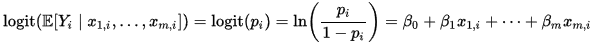If we exponentiate both sides of the equation above and do a little rearranging, on the right-hand-side (RHS) we get the familiar logistic function: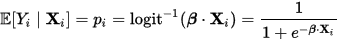One way to approach deriving the generalized logistic or softmax function for multinomial logistic regression is to start by having one logit linked linear predictor for each class K, plus some normalization factor to ensure that the total sum of the probabilities over all classes equals to one. This resulting system of equations is a system of log-linear probabilistic models:The ln(Z) term in the above system of equations is the (log of the) normalization factor, and is known as the partition function. As we are dealing with multinomial regression, this system of equations gives probabilities which are categorically distributed: Yᵢ | x ~ Categorical(p).

Exponentiating both sides and imposing the constraint: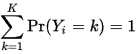givesThe RHS of the equation above is called the Gibbs measure and connects the softmax function to statistical mechanics. Next, solving for Z gives: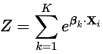And finally the system of equations becomes:The ratio on the RHS of each equation is the softmax function. In general, the softmax function is defined as: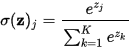for j = 1 … K. We can see that the softmax function normalizes a dimensional vector of arbitrary real values into a dimensional vector σ(z) whose components sum to 1 (in other words, a probability vector), and it also provides a weighted average of each zⱼ relative to the aggregate of zⱼ’s in a way that exaggerates differences (returns a value close to 0 or 1) if the zⱼ’s are very different from each other in terms of scale, but returns a moderate value if zⱼ’s are relatively the same scaleIt is desirable for a classifier model to learn parameters which give the former condition rather than the latter (i.e decisive vs indecisive).

Finally, just as the logit function is the link function for simple logistic regression and the logistic function is the inverse of the logit function, the multinomial logit function is the link function for multinomial logistic regression and the softmax can be thought of as the inverse of the multinomial logit function. Typically in multinomial logistic regression, maximum a-posterior (MAP) estimation is used to find the parameters β for each class k.

### Cross-Entropy and Ensemble Neural Network Classifier

Now that we have seen where the softmax function comes from, it’s time for us to use them in our neural net classifier models. The loss function to be minimized on softmax output layer equipped neural nets is the cross-entropy loss: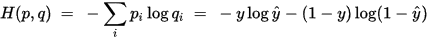Assuming p and q are discrete distributions

where is the true label for some iteration and ŷ is the neural network output at iteration i. This loss function is in fact the same one used for simple and multinomial logistic regression. The general definition of the cross-entropy function is: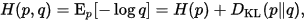The cross-entropy between and q is defined as the sum of the information entropy of distribution p, where is some underlying true distribution (in this case would be the categorical distribution of true class labels) and the Kullback–Leibler divergence of the distribution which is our attempt at approximating and itselfOptimizing over this function minimizes the information entropy of p (giving more certain outcomes in p) while at the same time minimizes the ‘distance’ between and q.

A theoretical treatment of using the softmax in neural nets as the output layer activation is given in Bridle’s article. The gist of the article is that using the softmax output layer with the neural network hidden layer output as each zⱼ, trained with the cross-entropy loss gives the posterior distribution (the categorical distribution) over the class labels. In general deep neural nets can vastly outperform simple and multinomial logistic regression at the expense of not being able to provide statistical significance of the features/parameters, which is a very important aspect of inference or finding out which features affect the outcome of the classification. The complete neural network is optimized using a robust optimizer of choice; RMSprop is usually a good start.

So now we’ll whip up a deep feedforward neural net classifier using the Keras functional API and do some wine classification. We’ll use an ensemble model of several neural nets to give us a robust classification (in practice this is what you should do, the variances in predictions of individual neural nets due to random initialization and stochastic gradient training must be averaged out for good results). The output of the ensemble model should give a vector of probabilities that some test example will belong to each class, i.e. a categorical distribution over the class labels.

One way to aggregate the results of each individual neural net model is to use a softmax at the ensemble output to give a final probability. In order to automatically determine the optimal weighting of the final softmax averaging, we’ll tack on another layer ‘gluing together’ the outputs of each individual neural net in the ensemble. A diagram of the architecture is below.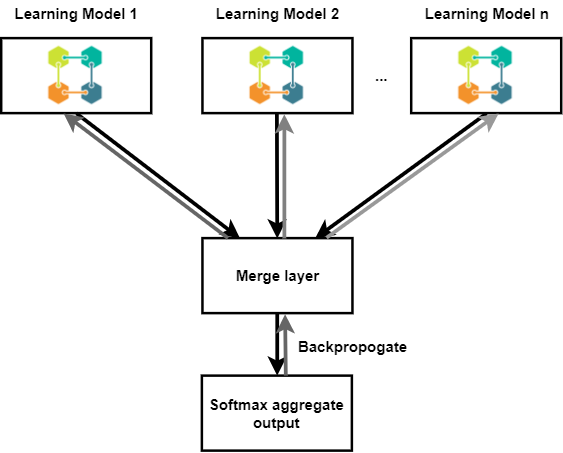Everyone loves block diagrams.

Each learning model will be differentiable from the final softmax aggregate output backwards. We can merge each of the sub-networks together using the Keras concatenate-merge layerThe concatenate layer concatenates the output tensors from each sub-network and allows the optimizer to optimize over the merged model. To simplify our training, each learning model will be trained on the same dataset. Bootstrapped sub-sets can be used but this makes it more complicated to train, as we would have to train each sub-network individually on its own input and target pair while freezing training updates on the rest of the learning models.

``````
import numpy as np
from sklearn.preprocessing import MinMaxScaler, OneHotEncoder
from keras.layers import Dense, Input, concatenate, Dropout
from keras.models import Model
from keras.optimizers import rmsprop

ensemble_num = 10 # number of sub-networks
bootstrap_size = 0.8 # 80% size of original (training) dataset
training_size = 0.8 # 80% for training, 20% for test

num_hidden_neurons = 10 # number of neurons in hidden layer
dropout = 0.25 # percentage of weights dropped out before softmax output (this prevents overfitting)

epochs = 200 # number of epochs (complete training episodes over the training set) to run
batch = 10 # mini batch size for better convergence

# get the holdout training and test set
temp = []
scaler = MinMaxScaler()
one_hot = OneHotEncoder() # one hot encode the target classes
dataset['data'] = scaler.fit_transform(dataset['data'])
dataset['target'] = one_hot.fit_transform(np.reshape(dataset['target'], (-1,1)) ).toarray()
for i in range(len(dataset.data)):
temp.append([dataset['data'][i], np.array(dataset['target'][i])])

# shuffle the row of data and targets
temp = np.array(temp)
np.random.shuffle(temp)
# holdout training and test stop index
stop = int(training_size*len(dataset.data))

train_X = np.array([x for x in temp[:stop,0]])
train_Y = np.array([x for x in temp[:stop,1]])
test_X = np.array([x for x in temp[stop:,0]])
test_Y = np.array([x for x in temp[stop:,1]])

# now build the ensemble neural network
# first, let's build the individual sub-networks, each
# as a Keras functional model.
sub_net_outputs = []
sub_net_inputs = []
for i in range(ensemble_num):
# two hidden layers to keep it simple
# specify input shape to the shape of the training set
net_input = Input(shape = (train_X.shape,))
sub_net_inputs.append(net_input)
y = Dense(num_hidden_neurons)(net_input)
y = Dense(num_hidden_neurons)(y)
y = Dropout(dropout)(y)
sub_net_outputs.append(y) # sub_nets contains the output tensors

# now concatenate the output tensors
y = concatenate(sub_net_outputs)

# final softmax output layer
y = Dense(train_Y.shape, activation='softmax')(y)

# now build the whole funtional model
model = Model(inputs=sub_net_inputs, outputs=y)
model.compile(optimizer='rmsprop', loss='categorical_crossentropy')

print("Begin training...")

# train the model
model.fit( [train_X] * ensemble_num, train_Y,validation_data=[ [test_X] * ensemble_num, test_Y],
epochs=epochs, batch_size=batch)

```
```

It can be seen from the results of training that the fancy wines are no match for our ensemble classifier. As well, feature selection is not terribly important for our model, as it learns the dataset quite well using all features. The training and validation losses become small to the order of 10^-5 and 10^-3 respectively after 200 epochs, and this indicates our ensemble neural net model is doing a good job of fitting the data and predicting on the test set. The output probabilities are nearly 100% for the correct class and 0% for the others.

### Conclusion:

In this article, we derived the softmax activation for multinomial logistic regression and saw how to apply it to neural network classifiers. It is important to remember to be careful when interpreting neural network outputs are probabilities. We then built an ensemble neural net classifier using the Keras functional API. Anyways, have fun running the code and as always please don’t hesitate to ask me about anything.